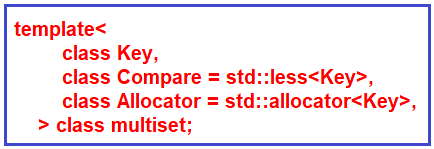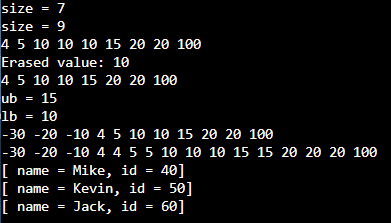# MultiSet in C++

## MultiSet in C++ with Examples

In this article, I am going to discuss Multiset in C++ with Examples. Please read our previous article, where we discussed Set in C++ with Examples. If you have not read our previous articles on STL, we highly recommend that you read them all as we have used concepts from the previous articles.

##### MultiSet in C++:

In the set, we have seen that we cannot insert duplicate elements. Let us say we have {1, 3, 6, 3, 6, 2} elements. These are 6 elements but only 4 will be inserted because 3 and 6 are duplicate elements. In the multiset, this is not the case. As we have 6 elements (including duplicates), all of them will be inserted in a multiset. If you print the multiset then these elements will be printed in the following order:

1, 2, 3, 3, 6, 6

The elements will be sorted. By default, it is std::less so it will sort in this order (ascending order). We can change the sort by changing it to std::greater. It will print in descending order. If you are using an object of your custom class, you can define your own custom function to define your sorting criteria for that object.

So, a major difference between these two is that set does not allow duplicates but multiset allows duplicates.

• A multiset is an associative container that contains a sorted set of unique objects of type key.
• A multiset is already included in the set header file so you do not have to include any other header file.
• In multiset, a user-provided compare can be supplied to change the ordering (sorting) if you are working with custom classes (user-defined classes).
• Search, removal, and insertion operations will take logarithmic time.
• It is usually implemented as RB (Red-Black) Tree:Here, we have a comparator std::less. We can provide different built-in comparators or user-defined comparators. Std::allocator is the default allocator. Allocators are objects responsible for encapsulating memory management.

##### MultiSet Functions in C++:
1. Size () – This function is used to find the number of elements present in the multiset container.
2. Equal (=) – it is used to assign multiset.
3. Clear () – it will remove all the elements from the multiset container.
4. Count (), find () – the count function will count the number of elements with a specific key. The find function will return an iterator to the element if it is present. For example, suppose we have a multiset m, m.find (3) so it will return the iterator to 3. In multiset, if we have duplicate values then the iterator will be on the lower bound. The lower bound means ‘>=’ and the upper bound is ‘<’.
5. Contains (key) – It is present in C++20. It is of Boolean type. It will check the presence of the given key.
6. Empty () – it will check to return whether the multiset is empty or not.
7. upper_bound (key), lower_bound (key) – Lower bound is ‘>=’ and upper bound is ‘<’.
8. Insert () and erase () – we will see these functions in detail.
##### Insert MultiSet Functions in C++:
1. Insert (Key key): It may not inset always. Let’s say we have a set, s = {1, 3, 2, 3, 6, 1}. Here, 1 is already present so insert (Key key) will not insert 1 there. But in the case of multiset, it will always insert even if that element is present it will insert that again. Now suppose we have a multiset, m = {1, 2, 3, 4, 4, 4, 5} and we want to insert 4 so where it will insert 4? It will insert at the upper bound. The Upper bound for this is 5 so it will insert 4 before 5. Now m = {1, 2, 3, 4, 4, 4, 4, 5}.
2. Insert (iterator pos1, iterator pos2): Here, we can pass two iterator positions. Let’s say you have a vector of elements, V = {_ _ _ x _ _ _ y _ _ _ _ }; You want to insert from position x to position y (excluding y) and these are iterator positions. It is like range [pos1, pos2). So, the close interval at pos1 and an open interval at pos2. This function will not return anything.
3. Insert (initializer_list l): Similarly, we can pass the initializer list. You can think of it as in the above example if we are inserting from pos1 to pos2, so it will be an initializer list or you can say a subset of the vector. So, you will pass that initializer list and it will add all the elements to the multiset. It will also not return anything. We will see examples of all of these functions.
##### Erase MultiSet Functions in C++:
1. erase (iterator pos): It removes the element at the given position and returns the iterator following the last element removed.
2. erase (iterator pos1, iterator pos2): As we have seen in the insert function, we can also pass two positions in this function so that all the elements between these positions will be removed. So pos1 will be included and pos2 will be excluded. It also returns the iterator following the last element removed.
3. erase (key): In this signature, we have passed the iterator to the key but here we are passing the key itself. If any key is present in the multiset, it will remove all the elements with the key values. It will return the number of elements removed.

Now let’s see the complete program.

##### Example to Understand the MultiSet Container in C++:
```#include <iostream>
#include <set>
#include <vector>
using namespace std;

class Student{
public:
int id;
string name;

void print_student() const {
cout << "[ name = " << name << ", id = " << id << "]\n";
}

bool operator < (const Student& other) const {
return (this->id < other.id);
}
};

int main() {
multiset<int> ms = {10, 20, 5, 10, 15, 20, 4};
cout << "size = " << ms.size() << endl;
ms.insert(100);
ms.insert(10);
cout << "size = " << ms.size() << endl;

for(auto& el: ms)
cout << el << " ";
cout << endl;

auto it = ms.erase(ms.find(10));
cout << "Erased value: " << *it << endl;

//int num_erased = s.erase(10);
//cout << "num_erased = " << num_erased << endl;

for(auto& el: ms)
cout << el << " ";
cout << endl;

auto ub = ms.upper_bound(10);
auto lb = ms.lower_bound(10);
cout << "ub = " << *ub << endl;
cout << "lb = " << *lb << endl;

ms.insert({-10, -30, -20});
for(auto& el: ms)
cout << el << " ";
cout << endl;

vector<int> v = {10, 20, 15, 5, 4};
ms.insert(v.begin(), v.end());
for(auto& el: ms)
cout << el << " ";
cout << endl;

//------------
multiset<Student> sst = {{60, "Jack"}, {40, "Mike"}, {50, "Kevin"}};
for(auto& st: sst)
st.print_student();

return 0;
}
```
###### Output: